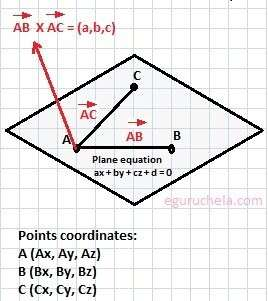# Cartesian Equation of Plane Calculation

Calculate the equation of a three-dimensional plane in space for entered values.

Please enter the coordinates of :

 Point A : Ax Ay Az

 Point B : Bx By Bz

 Point C: Cx Cy Cz

 Equation of the plane: x y z =0

Calculator find the equation of a three-dimensional plane in space, suppose the normal vector is defined as:
n = < A, B, C>

where :
n is a vector,
and A, B, and C are scalars.There are different methods to define a plan like; a point and normal vector, three non-collinear points, a line and a point not on the line, two parallel and non-coincident line and two intersecting lines.

The Cartesian equation of a plane is ax + by + cy + d = 0 where a,b and c are the vector normal to the plane. A Cartesian coordinate system for three-dimensional space plane has three axis(x, y, and z).

We can determine the equation of the plane that contains the 3 point in the xyz-coordinate in following form:

ax + by + cz + d = 0

where a, b, c, and d are real numbers and derivation is as follows:

a = (By-Ay)(Cz-Az)-(Cy-Ay)(Bz-Az)

b = (Bz-Az)(Cx-Ax)-(Cz-Az)(Bx-Ax)

c = (Bx-Ax)(Cy-Ay)-(Cx-Ax)(By-Ay)

d = -(aAx+bAy+cAz).

Let's take the example where all the 3 points are as follows in three-dimensional space: